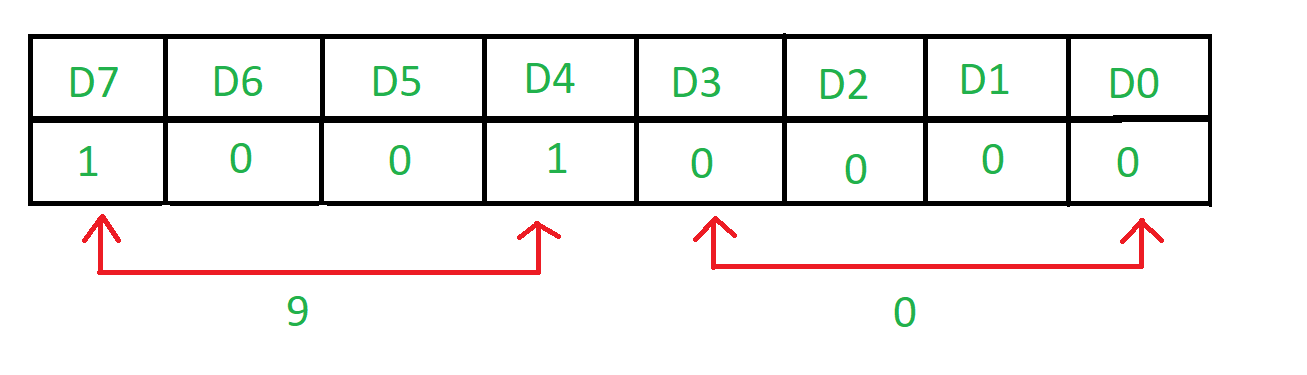GeeksforGeeks App
Open AppBrowser
Continue

# Assembly program to transfer the status of switches

Problem – Write an assembly language program in 8085 of interfacing between 8085 and 8255. 8 switches are connected at port A. Transfer the status of these switches into port B where LEDs are connected. Example –Input port is A and output port is B. Algorithm –

1. Construct the control word register
2. Input the data from port A
3. Display the result in port B

Program –

Explanation –

1. MVI A, 92 means that the value of control register is 92.
```D7=1 as it is in I/O mode
D6=0 & D5=0 as Poet A is in m0 mode
D4=1 as Port A is taking input
D3=0 & D0=0 as Port C is not taking part
D2=0 as mode of Port B is m0
D1=0as Port B is displaying the result```
1. OUT 83 putting the value of A in 83H which is the port number of port control register.
2. IN 80 taking input from 80H which is the port number of port A.
3. OUT 81 displaying the result in 81H which is the port number of port B.
4. RET return

• The program is simple and straightforward, consisting of only two instructions.

• The program uses the input/output instruction to read the status of the switches, which is an efficient method.

• The program uses the store accumulator instruction to transfer the status of the switches to a memory location.

• The program can be easily modified to transfer the status of other input devices, such as sensors or buttons.